# Comparison of Distribution Functions

DistributionFunctional FormMeanStandard Deviation

### Binomial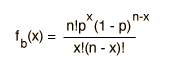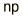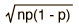### Gaussian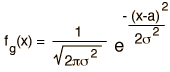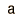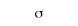### Poisson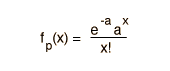### Compare the results of these distributions

Index

Applied statistics concepts

 HyperPhysics*****HyperMath*****Algebra R Nave
Go Back

# Binomial Distribution Function

 Distribution Functional Form Mean Standard Deviation BinomialThe binomial distribution function specifies the number of times (x) that an event occurs in n independent trials where p is the probability of the event occurring in a single trial. It is an exact probability distribution for any number of discrete trials. If n is very large, it may be treated as a continuous function. This yields the Gaussian distribution. If the probability p is so small that the function has significant value only for very small x, then the function can be approximated by the Poisson distribution.

If the probability of an event is p = and there are n = events, then the probability of that event being observed times is x 10^. For these conditions, the mean number of events is and the standard deviation is .

### Note!

This calculation must evaluate the factorials of very large numbers if the number of events is large. A sum of logarithms was used to evaluate the factorials, and use was made of the fact that there is a quotient of factorials which can be reduced. Still, the factorials can get too large. Use of the Gaussian distribution may be necessary for large values of n.

With the parameters as defined above, the conditions for validity of the binomial distribution are

• each trial can result in one of two possible outcomes, which could be characterized as "success" or "failure".
• the probability of "success", p, is constant from trial to trial.
• the trials are independent.

 Comments about the form of the function Example of throwing dice Specific example: binomial distribution
Index

Distribution functions

Applied statistics concepts

 HyperPhysics*****HyperMath*****Algebra R Nave
Go Back

When you examine the form of the binomial distribution function:it is a rather formidable collection of symbols and factorials. These terms arise from an examination of the probability of discrete events. Perhaps the following summary will be helpful: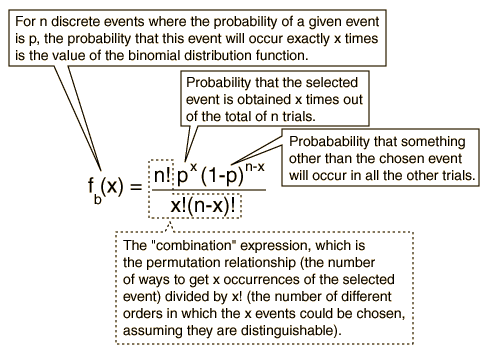Permutations Combinations Basic Probability
Index

Applied statistics concepts

 HyperPhysics*****HyperMath*****Algebra R Nave
Go Back